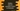# Python program to convert Unicode or ASCII value to a character## Convert Unicode or ASCII value to a character using python :

In this python programming tutorial, we will learn how to convert a Unicode value to its character value. The program will take one Unicode value from the user and it will print the character that it represents.

Unicode 11 contains around 137,439 characters. ASCII has 128 _values in total. The ASCII value of a character is the same as its Unicode value. So, you can use the same process we are showing in this example to convert an _ASCII value to its character representation.

## char() method :

Python comes with one inbuilt method to convert one Unicode value to its string representation. The method is defined as below :

``chr(i)``

As you can see, this method takes one integer as a parameter and returns the string representation of the integer.

For example, the value of 97 will be ‘a’.

Its argument lies in 0 through 1,114,111. If the argument is not in this range, it will throw one ValueError.

### Python program :

``````u = int(input("Enter the unicode value : "))

print("The string representation of {} is {}".format(u,chr(u)))``````

In this program, the user input is stored in the variable_ u_. Then, we are converting this value to its character representation using _chr _method.

### Sample Output :

``````Enter the unicode value : 65
The string representation of 65 is A

Enter the unicode value : 69
The string representation of 69 is E

Enter the unicode value : 101
The string representation of 101 is e

Enter the unicode value : 200
The string representation of 200 is È

Enter the unicode value : 345
The string representation of 345 is ř

Enter the unicode value : 999
The string representation of 999 is ϧ``````